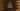# C++ program to print a Pascal's triangle## Pascal’s triangle:

Pascal’s triangle is a number triangle. Each number is the sum of two numbers above it. This is named after French Mathematician Blaise Pascal. This is an equilateral triangle. Printing Pascal’s triangle in C++ is one of the most common problem you will find in your exam or in a programming interview. In this post, we will learn how to create a Pascal triangle of any height in C++. Our program will take the height of the triangle as user input and it will print the triangle.

The two red lines indicates the sum of two numbers. For example, third line middle element 2 is the sum of first and second 1 of the second line.

### C++ program:

Below is the complete C++ program:

``````#include <iostream>
using namespace std;

void pascalTriangle(int n)
{
string blank = " ";

for (int i = 1; i <= n; i++)
{
int currentValue = 1;

for (int j = 1; j < (n - i + 1); j++)
{
cout << blank;
}

for (int j = 1; j <= i; j++)
{
cout << currentValue << blank;
currentValue = currentValue * (i - j) / j;
}

cout << "\n";
}
}

int main()
{
int height;
cout << "Enter the height of the triangle :" << endl;
cin >> height;
pascalTriangle(height);
}``````

### Explanation:

In this program,

• we are asking the user to enter the height of a triangle. This value is stored in the variable height.
• We are calling pascalTriangle method with the value of height. This method prints the pascal triangle.
• We have one for loop in this mehod and two more inner for loops inside this for loop.
• The outer for loop runs to print the rows of the triangle. So, it will run for height number of times.
• The first inner loop is used to print the blank spaces at the start of each row. And, the second loop is used to print the numbers. We are printing one new line at the end of each row.

### Sample output:

``````Enter the height of the triangle :
5
1
1 1
1 2 1
1 3 3 1
1 4 6 4 1 ``````